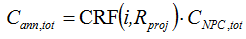# HOMER Grid 1.8Type: Output Variable Units: \$/year Symbol: Cann,tot

The total annualized cost is the annualized value of the total net present cost. HOMER calculates the total annualized cost using the following equation:where: CNPC,tot = the total net present cost [\$] i = the annual real discount rate [%] Rproj = the project lifetime [yr] CRF() = a function returning the capital recovery factor

HOMER uses the total annualized cost to calculate the levelized cost of energy.